Build a DFA to accept Binary strings that starts or ends with “01”

• Last Updated : 26 Nov, 2021

Given binary string str, the task is to build a DFA that accepts the string if the string either starts with “01” or ends with “01”.

Input: str = “010000”
Output: Accepted
Explanation:
The given string starts with “01”.

Input: str = “1100111”
Output: Not Accepted
Explanation:
The given string neither starts with nor ends with “01”.

DFA or Deterministic Finite Automata is a finite state machine that accepts a string(under some specific condition) if it reaches a final state, otherwise rejects it.
In DFA, there is no concept of memory, therefore we have to check the string character by character, beginning with the 0th character. The input set of characters for the problem is {0, 1}. For a DFA to be valid, there must a transition rule defined for each symbol of the input set at every state to a valid state. Therefore, the following steps are followed to design the DFA:

• In this case, the strings that start with 01 or end with 01 or both start with 01 and end with 01 should be acceptable.
• Make an initial state and transit its input alphabets, i.e, 0 and 1 to two different states.
• Check for acceptance of string after each transition to ignore errors.
• First, make DfA for minimum length string then go ahead step by step.
• Define Final State(s) according to the acceptance of string.

Step by Step Approach to design a DFA:

• Step 1: Make an initial state “A”. The minimum possible string is 01 which is acceptable. For this, make the transition of 0 from state “A” to state “B” and then make the transition of 1 from state “B” to state “C” and notice this state “C” as the final state.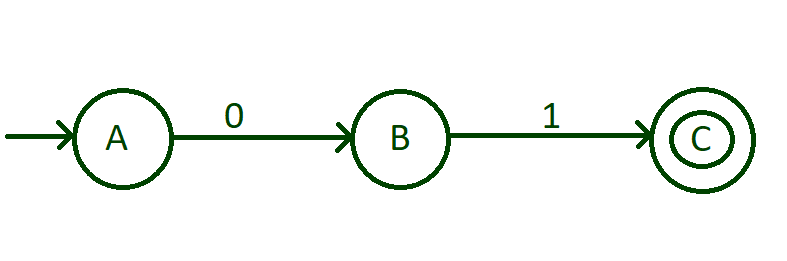• Step 2: Now, we have designed the DFA that start with 01. To accept all the strings that start with 01 like 011, 010, 010000, 01111, 010101000010001 etc, we need to put a self-loop of 0 and 1 to the state “C”. This self-loop contains all combination of 0 and 1.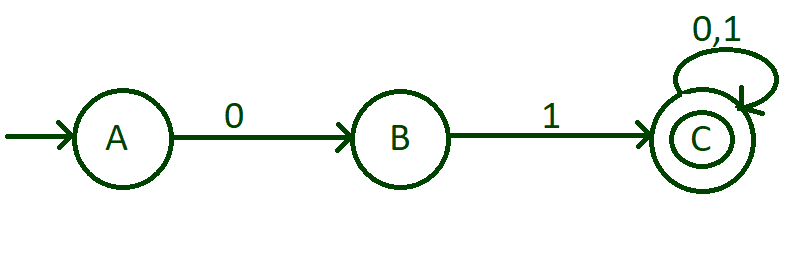• Step 3: Now, we need to think about the string that ends with “01”. We have done transition of 0 of state “A”, then with input 1 of the state “A”. With this minimum possible string that ends with 01 is 101. For this, do the transition of input 1 of state “A” to state “D” then do the transition of input 0 from the state “D” to state “E” and then do the transition of input 1 from state “E” to state “F” and notice this “F” state as the final state.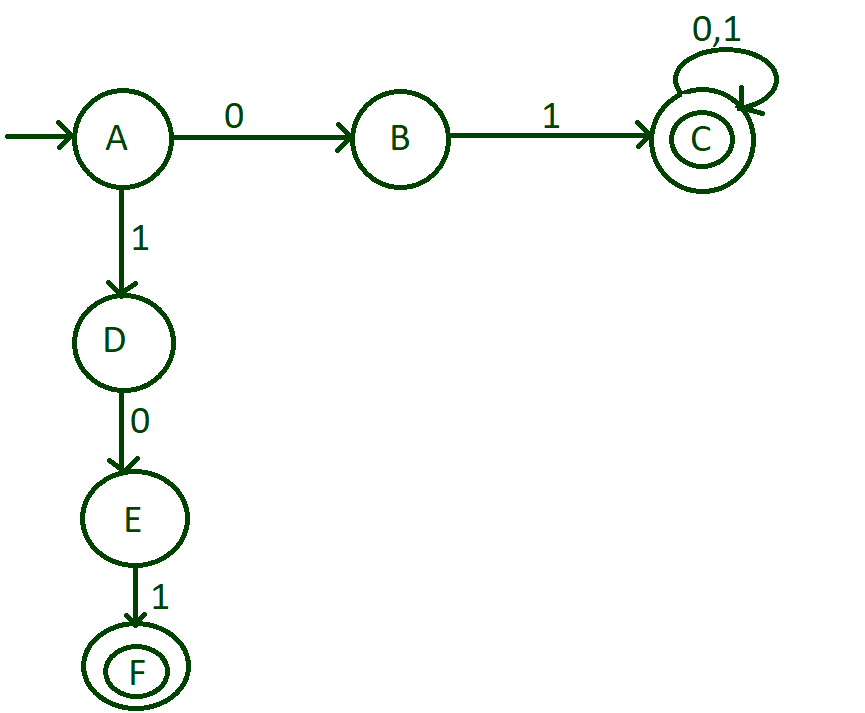• Step 4: There is one more possibility that any number of 1’s comes in beginning and then end with 01. For this, make a self-loop of 1 on state “D” and any number of zeros can come before 1 that comes in the end. For this, put a self-loop of 0 and state “E”.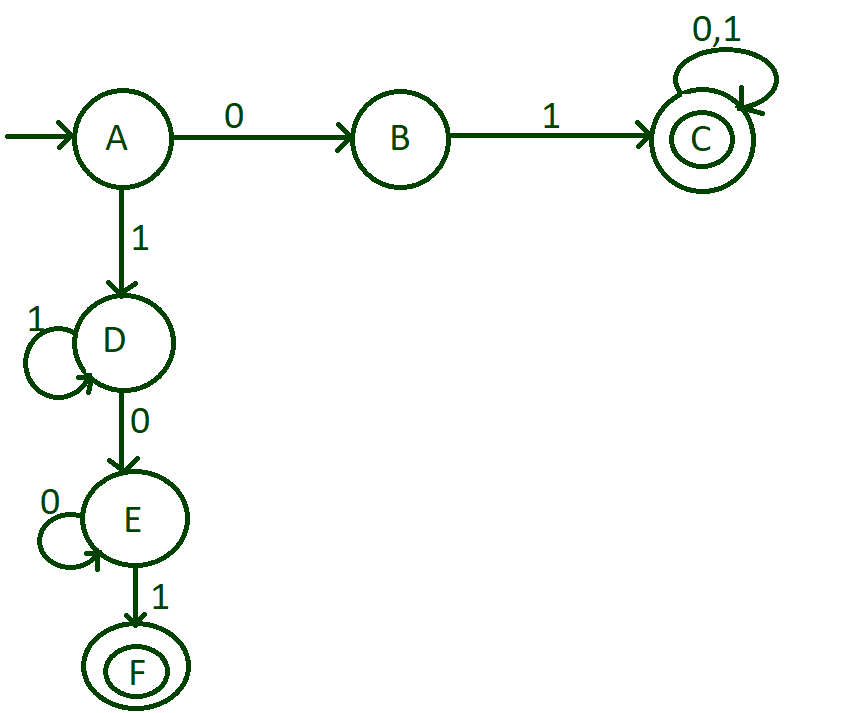• Step 5: Till now we have done with the strings that start with 1 and end with 01. Now, we need to think about the string that starts with 0 and ends with 01. For this, make a transition of input 0 from state “B” to state “E”.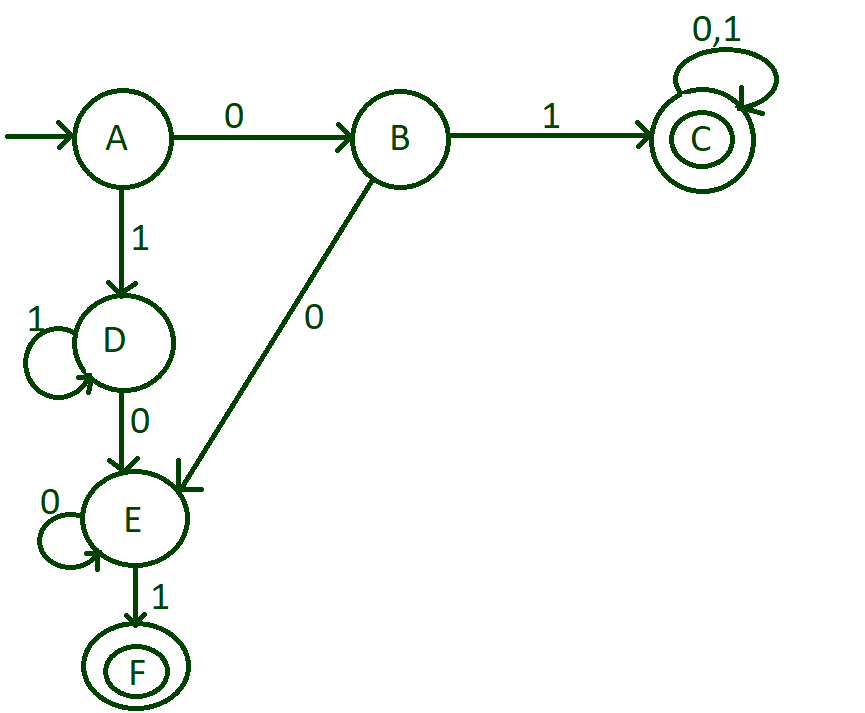• Step 6: Now we are just left with the input alphabets of the state “F”. Transit input 1 from state “F” to state “D” and then make transit input 0 from state “F” to state “E”.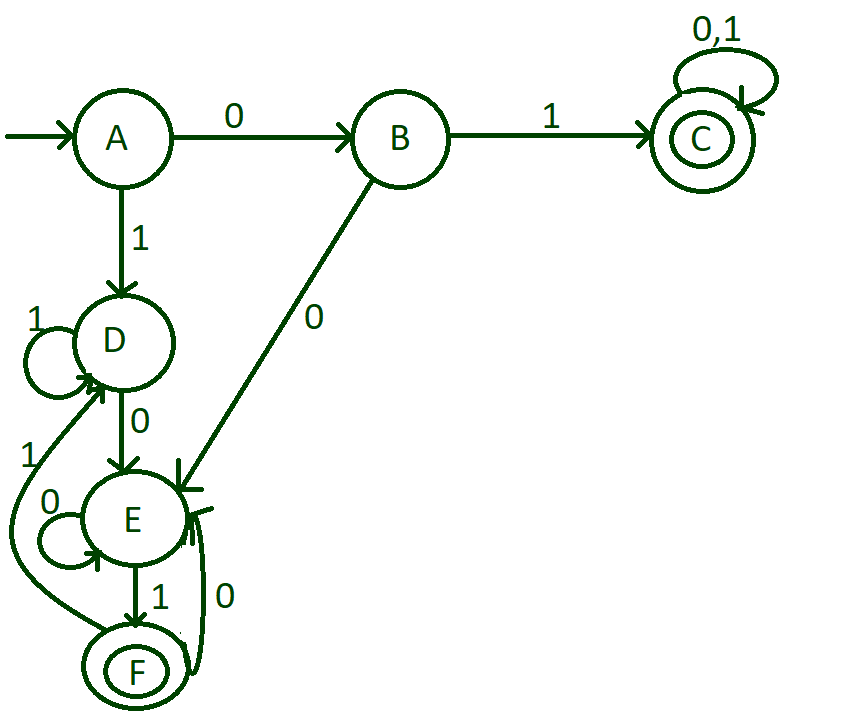Transition table and Transition rules of the above DFA: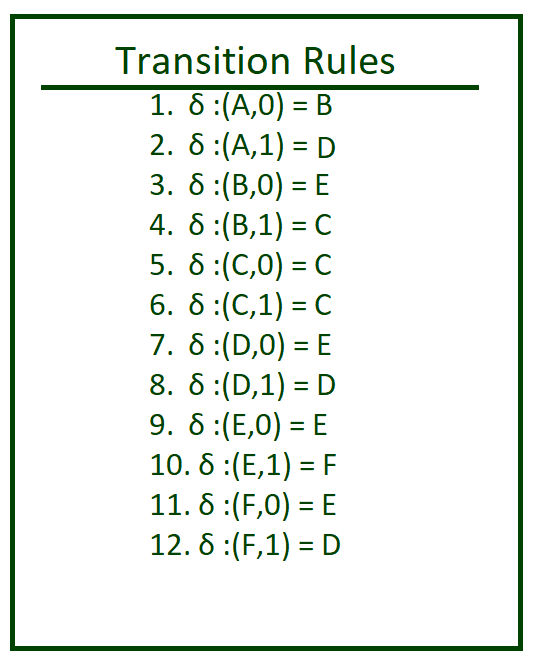Below is the implementation of the above approach:

C++

 // C++ program to check if a string// either starts or ends with 01#includeusing namespace std; void stateA(string);void stateB(string);void stateC(string);void stateD(string);void stateE(string);void stateF(string); // Function for transition// state Avoid checkstateA(string n){         // State transition to    // B if the character is    // 0    if(n == '0')       stateB(n.substr(1));            // State transition to    // D if the character is    // 1    else       stateD(n.substr(1));} // Function for transition// state B        void stateB(string n){         // Check if the string has    // ended    if (n.length() == 0)        cout << "string not accepted";    else    {                 // State transition to C        // if the character is 1        if(n == '1')            stateC(n.substr(1));         // State transition to D        // if the character is 0        else            stateD(n.substr(1));    }    } // Function for transition// state Cvoid stateC(string n){    cout << "String accepted";} // Function for transition// state Dvoid stateD(string n){    if (n.length() == 0)        cout << "string not accepted";    else    {                 // State transition to D        // if the character is 1        if (n == '1')            stateD(n.substr(1));         // State transition to E        // if the character is 0        else            stateE(n.substr(1));    }} // Function for transition// state Evoid stateE(string n){    if (n.length() == 0)        cout << "string not accepted";    else    {                 // State transition to E        // if the character is 0        if(n == '0')            stateE(n.substr(1));         // State transition to F        // if the character is 1        else            stateF(n.substr(1));    }} // Function for transition// state Fvoid stateF(string n){    if(n.length() == 0)        cout << "string accepred";    else    {                 // State transition to D        // if the character is 1        if(n == '1')            stateD(n.substr(1));         // State transition to E        // if the character is 0        else            stateE(n.substr(1));    }} // Driver codeint main(){    string n = "0100101";         checkstateA(n);    return 0;} // This code is contributed by chitranayal

Java

 // Java program to check if a string// either starts or ends with 01import java.util.*; class GFG{     // Function for transition// state Astatic void checkstateA(String n){         // State transition to    // B if the character is    // 0    if (n.charAt(0) == '0')       stateB(n.substring(1));            // State transition to    // D if the character is    // 1    else       stateD(n.substring(1));} // Function for transition// state B        static void stateB(String n){         // Check if the string has    // ended    if (n.length() == 0)        System.out.println("string not accepted");    else    {                 // State transition to C        // if the character is 1        if (n.charAt(0) == '1')            stateC(n.substring(1));         // State transition to D        // if the character is 0        else            stateD(n.substring(1));    }    } // Function for transition// state Cstatic void stateC(String n){    System.out.println("String accepted");} // Function for transition// state Dstatic void stateD(String n){    if (n.length() == 0)        System.out.println("string not accepted");    else    {                 // State transition to D        // if the character is 1        if (n.charAt(0) == '1')            stateD(n.substring(1));         // State transition to E        // if the character is 0        else            stateE(n.substring(1));    }} // Function for transition// state Estatic void stateE(String n){    if (n.length() == 0)       System.out.println("string not accepted");    else    {                 // State transition to E        // if the character is 0        if(n.charAt(0) == '0')            stateE(n.substring(1));         // State transition to F        // if the character is 1        else            stateF(n.substring(1));    }} // Function for transition// state Fstatic void stateF(String n){    if (n.length() == 0)        System.out.println("string accepred");    else    {                 // State transition to D        // if the character is 1        if (n.charAt(0) == '1')            stateD(n.substring(1));         // State transition to E        // if the character is 0        else            stateE(n.substring(1));    }} // Driver Codepublic static void main(String args[]){    String n = "0100101";         checkstateA(n);}} // This code is contributed by jyoti369

Python3

 # Python3 program to check if# a string either starts or# ends with 01 # Function for transition# state Adef checkstateA(n):     # State transition to    # B if the character is    # 0    if(n=='0'):        stateB(n[1:])     # State transition to    # D if the character is    # 1    else:        stateD(n[1:]) # Function for transition# state B        def stateB(n):     # Check if the string has    # ended    if (len(n)== 0):        print("string not accepted")    else:                # State transition to C        # if the character is 1        if(n=='1'):            stateC(n[1:])         # State transition to D        # if the character is 0        else:            stateD(n[1:])          # Function for transition# state C   def stateC(n):    print("String accepted")  # Function for transition# state Ddef stateD(n):    if (len(n)== 0):        print("string not accepted")    else:            # State transition to D        # if the character is 1        if (n=='1'):            stateD(n[1:])         # State transition to E        # if the character is 0        else:            stateE(n[1:])  # Function for transition# state Edef stateE(n):    if (len(n)== 0):        print("string not accepted")    else:           # State transition to E        # if the character is 0        if(n=='0'):            stateE(n[1:])         # State transition to F        # if the character is 1        else:            stateF(n[1:])  # Function for transition# state Fdef stateF(n):    if(len(n)== 0):        print("string accepred")    else:         # State transition to D        # if the character is 1        if(n=='1'):            stateD(n[1:])         # State transition to E        # if the character is 0        else:            stateE(n[1:])      # Driver codeif __name__ == "__main__":    n = "0100101"    checkstateA(n)

C#

 // C# program to check if// a string either starts// or ends with 01using System;using System.Collections;using System.Collections.Generic;class GFG{     // Function for transition// state Astatic void checkstateA(string n){  // State transition to  // B if the character is  // 0  if(n == '0')    stateB(n.Substring(1));   // State transition to  // D if the character is  // 1  else    stateD(n.Substring(1));}  // Function for transition// state B        static void stateB(string n){      // Check if the string has  // ended  if (n.Length == 0)  {    Console.Write("string not accepted");  }  else  {    // State transition to C    // if the character is 1    if(n == '1')      stateC(n.Substring(1));     // State transition to D    // if the character is 0    else      stateD(n.Substring(1));  }    } // Function for transition// state Cstatic void stateC(string n){  Console.Write("string accepted");}  // Function for transition// state Dstatic void stateD(string n){  if (n.Length == 0)    Console.Write("string not accepted");  else  {     // State transition to D    // if the character is 1    if (n == '1')      stateD(n.Substring(1));     // State transition to E    // if the character is 0    else      stateE(n.Substring(1));  }}  // Function for transition// state Estatic void stateE(string n){  if (n.Length == 0)    Console.Write("string not accepted");  else  {     // State transition to E    // if the character is 0    if(n == '0')      stateE(n.Substring(1));     // State transition to F    // if the character is 1    else      stateF(n.Substring(1));  }}  // Function for transition// state Fstatic void stateF(string n){  if(n.Length == 0)    Console.Write("string accepted");  else  {    // State transition to D    // if the character is 1    if(n == '1')      stateD(n.Substring(1));     // State transition to E    // if the character is 0    else      stateE(n.Substring(1));  }} // Driver codepublic static void Main(string []args){  string n = "0100101";  checkstateA(n);}} // This code is contributed by rutvik_56

Javascript


Output:
String accepted

Time Complexity: O(N)
Auxiliary Space: O(N)

My Personal Notes arrow_drop_up